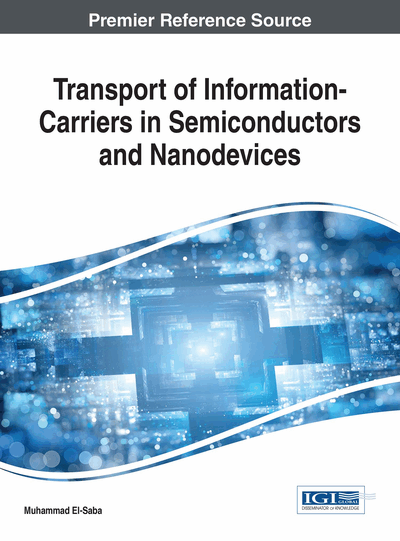# Quantum Transport Theory of Charge Carriers

DOI: 10.4018/978-1-5225-2312-3.ch004
Available
\$33.75
List Price: \$37.50
10% Discount:-\$3.75
TOTAL SAVINGS: \$3.75

Top

## 1. Overview And Chapter Objectives

Although the Schrödinger formalism is the de-facto standard, other possible approaches to quantum mechanics are available. The quantum transport theory, which is based on the statistical quantum mechanics, is the most rigorous method describing the transport phenomena in solids. The basis of the quantum transport theory is as strong as that of the statistical mechanics of systems in equilibrium, so that the BTE itself can be deduced from it! Indeed, different but mathematically equivalent formulations have eventually been developed, with their respective advantages and disadvantages, among which we have the significant works of Wigner, Feynman and Keldysh. Interestingly enough, these approaches are not based on the concept of wave-functions, but on rather different mathematical objects such as quasi-distribution functions (WDF), path integrals (Feynman), nonequilibrium Green functions (NEGF), and still they provide the very same predictions as the Schrödinger equation. In fact, these approaches are capable of handling both quantum-coherent propagation and dissipative scattering effects. Actually, there exist several approaches in the quantum transport theory, among them one can cite:

• Density matrix formulation,

• Wigner distribution function,

• Feynman path integral,

• Green’s Functions (Kadanoff-Baym & Keldish) approaches.

These quantum approaches may be different in mathematical formalism, but a transform is always available to go from formalism to another and vice versa. The hierarchy of quantum transport models, for both ballistic and dissipative systems, is shown in Figure 1.

The Landauer-Büttiker approach describes the conductance of quantum system in the ballistic (collision-less) regime, where carriers are not scattered. The non-equilibrium Green's function (NEGF) formalism can handle open quantum systems as well as external contacts. The method can be used in both ballistic and a scattering dominated regime. Under ballistic conditions, the NEGF method is mathematically equivalent to solving the Schrödinger equation with open boundary conditions. There are two basic NEGF formulations, namely; the Kadanoff-Baym (K-B) approach and the Keldysh method. The K-B approach (1970) has been extended by Mahan and Hansch (1983). Nowadays this approach is sometimes referred to the quantum Boltzmann transport equation (QBTE). The so-called Contact-Block Reduction (CBR) method is an approximate method to solve the Schrodinger equation self-consistently with Poisson’s equation in open systems (Mamaluy et al., 2005). The CBR may be considered as a variant of the NEGF for ballistic transport, using an equilibrium distribution function.

The Wigner Boltzmann transport equation (WBTE) can also be used in both ballistic and scattering dominated regimes. However, the WBTE is less or more similar to the NEGF method.

The quantum Liouville (or Liouville von Neumann) equation describes the quantum evolution of the density matrix and forms the fundamental equation of quantum transport. The so-called Pauli Master equation (PME) is derived from the quantum Liouville equation. The Pauli master equation is a frequently used to describe irreversible processes in quantum systems and can be also used in the ballistic and scattering-dominated regimes. The Lindblad master equation1 is a generalized form of the Liouville equation. It describes the non-unitary evolution of the density matrix of a system.

## Complete Chapter List

Search this Book:
Reset